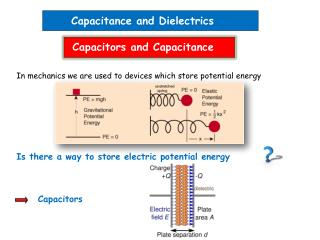DownloadDownload PresentationCapacitance and Dielectrics

# Capacitance and Dielectrics

Download Presentation## Capacitance and Dielectrics

- - - - - - - - - - - - - - - - - - - - - - - - - - - E N D - - - - - - - - - - - - - - - - - - - - - - - - - - -
##### Presentation Transcript

1. Capacitance and Dielectrics Capacitors and Capacitance In mechanics we are used to devices which store potential energy Is there a way to store electric potential energy Capacitors

2. Any 2 conductors insulated from each other form a capacitor can be realized by an insulating materials (dielectric) or vacuum In circuit diagrams a capacitor is represented by the symbol: Let’s charge a capacitor -Q +Q Vab=Va-Vb Vb Va

3. Remember our electric field calculations for various charged objects We always find voltage Since Capacitance depends only on geometry and dielectric properties, not on charge SI unit 1F=1C/V read F=Farad in honor of Michael Faraday Capacitance is an intuitive characterization of a capacitor. It tells you: how much charge a capacitor can hold for a given voltage (potential difference). The more the higher the capacitance

4. Calculating capacitance According to the major task in calculating C is calculating Vab Homogeneous field, E=/0 for the limit d<< plate dimensions Parallel-plate capacitor ------------------------------------------- Using +++++++++++++++++++++++++ Charge density d We obtain the capacitance C of a parallel-plate capacitor in vacuum as Note: 1F is a huge capacitance. More typical values are 1µF=10-6F to 1pF=10-12F

5. Demonstration: parallel-plate capacitor

6. A few slightly more involved examples Capacitance of a spherical capacitor Step 1: calculate the electric field using Gauss’s law between the 2 spheres for Image from http://hyperphysics.phy-astr.gsu.edu/hbase/electric/capsph.html Step 2: calculate the voltage Vab for a given amount of charge Q on the spheres Step 3: applying

7. Clicker question Does an isolated (individual) charged sphere have capacitance? 1) No, where would the electric field lines end? 2) Yes, I just don’t know the value 3) Yes, it is a special case of in the limit b-> Image from http://hyperphysics.phy-astr.gsu.edu/hbase/electric/capsph.html

8. Capacitance per length of a cylindrical capacitor (coaxial cable) Step 1: calculate the electric field using Gauss’s law Cylinder with a<r<b L Step 2: calculate the voltage Vab Step 3: applying Typical value for antennas, VCRs 69pF/m